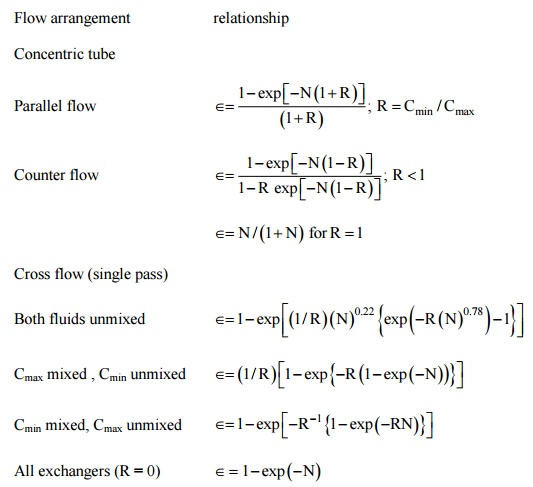Home | | Heat and Mass Transfer | NTU Method

# NTU Method

Heat Exchangers Effectiveness - Useful Parameters, Effectiveness - NTU Relations

NTU METHOD:

Heat Exchangers Effectiveness - Useful Parameters

In the design of heat exchangers, the efficiency of the heat transfer process is very important. The method suggested by Nusselt and developed by Kays and London is now being extensively used. The effectiveness of a heat exchanger is defined as the ratio of the actual heat transferred to the maximum possible heat transfer.

Let mh and mc be the mass flow rates of the hot and cold fluids, ch and cc be the respective specific heat capacities and the terminal temperatures be Th and T h for the hot fluid at inlet and outlet, Thi and Th0 for the cold fluid at inlet and outlet. By making an energy balance and assuming that there is no loss of energy to the surroundings, we writeFrom Eq. (10.13), it can be seen that the fluid with smaller thermal capacity, C, has the greater temperature change. Further, the maximum temperature change of any fluid would be

(Th i -Tci )and this Ideal temperature change can be obtained with the fluid which has the minimum heat capacity rate. Thus,Or, the effectiveness compares the actual heat transfer rate to the maximum heat transfer rate whose only limit is the second law of thermodynamics. An useful parameter which also measures the efficiency of the heat exchanger is the 'Number of Transfer Units', NTU, defined as

NTU = Temperature change of one fluid/LMTD.

Thus, for the hot fluid: NTU = (Th i  -Th0 )/LMTD , and

 for the cold fluid: NTU T -T / LMTDThe heat exchanger would be more effective when the NTU is greater, and therefore,

NTU = AU/Cmin (3.15)

An another useful parameter in the design of heat exchangers is the ratio the minimum to the maximum thermal capacity, i.e., R = Cmin/Cmax,

where R may vary between I (when both fluids have the same thermal capacity) and 0 (one of the fluids has infinite thermal capacity, e.g., a condensing vapour or a boiling liquid).

Effectiveness - NTU Relations

For any heat exchanger, we can write: Î=f (NTU, Cmin / Cmax ).In order to determine a specific form of the effectiveness-NTU relation, let us consider a parallel flow heat exchanger for which C min =Ch . From the definition of effectiveness (equation 10.14), we getHeat Exchanger Effectiveness RelationKays and London have presented graphs of effectiveness against NTU for Various values of R applicable to different heat exchanger arrangements,.Study Material, Lecturing Notes, Assignment, Reference, Wiki description explanation, brief detail
Mechanical : Heat and Mass Transfer : Phase Change Heat Transfer and Heat Exchangers : NTU Method |